## conference papersJOURNAL OFAPPLIEDCRYSTALLOGRAPHY
ISSN: 1600-5767
Volume 40| Part s1| April 2007| Pages s501-s505

## Mixed magnetic phase in 6H-type BaFeO3−δ

aResearch Reactor Institute, Kyoto University, Kumatori-cho, Sennan-gun, Osaka 590-0494, Japan, bInstitute of Materials Structure Science, High Energy Accelerator Research Organization, Tsukuba, Ibaraki 305-0801, Japan, and cGraduate School of Materials Science, University of Hyogo, 3-2-1 Kouto, Kamigori-cho, Ako-gun, Hyogo 678-1297, Japan
*Correspondence e-mail: kmori@rri.kyoto-u.ac.jp

(Received 16 August 2006; accepted 12 January 2007; online 9 February 2007)

The magnetic state in 6H-type BaFeO3−δ at low temperature was studied using small-angle neutron scattering, positive-muon spin relaxation and magnetization measurements. These experiments demonstrate the appearance of two different types of magnetic states: an antiferromagnetic ordering with a long-range correlation and magnetic domains with a short-range correlation. The antiferromagnetic Fe spin arrangement occurs below 130 K. In contrast, the magnetic domains are formed below 170 K and the average size of the magnetic domains was estimated as ~124 Å. These results explain the discrepancy of the Néel temperatures between three measurement techniques: magnetization, neutron powder diffraction and Mössbauer measurements. Furthermore, it was found that the magnetic domains coexist with the long-range antiferromagnetic ordering below 130 K.

### 1. Introduction

BaFeO3−δ is a perovskite-type iron oxide with a rare oxidation state of +4 (e.g. Van Hook, 1964; Gallagher et al., 1965; Macchesney et al., 1965). It is well known that BaFeO3−δ adopts many forms depending on the oxygen deficiency δ: triclinic (2.64 < δ < 2.67), rhombohedral (2.62 < δ < 2.64), tetragonal (2.75 < δ < 2.81) and hexagonal (2.63 < δ < 2.92) (Mori, 1966). Furthermore, the hexagonal phase is classified into 6H- and 12H-type BaFeO3−δ (e.g. Takeda et al., 1974; Grenier, Fournes et al., 1989; Grenier, Wattiaux et al., 1989; Parras et al., 1989). Among these phases, 6H-type BaFeO3−δ has attracted much attention because its electronic and magnetic states are very complicated.

It had long been believed that 6H-type BaFeO3−δ showed ferromagnetism below ca 250 K and a ferromagnetic to antiferromagnetic transition at around 160 K (Mori, 1970). However, recent Mössbauer and high-field magnetization measurements demonstrated that there was no magnetic ordering at temperatures between 160 and 220 K, that is, the magnetic state was paramagnetic (Iga, Nishihara, Kido & Takeda, 1992). In addition, it was speculated that the origin of the drastic increase in the magnetic susceptibility below 250 K is associated with charge disproportionation of Fe4+, 2Fe4+ → Fe(4+τ)+ + Fe(4−τ)+ (0 < τ ≤ 1), observed in CaFeO3−δ and Sr3Fe2O7−δ (e.g. Takano et al., 1977; Takeda et al., 1978; Dann et al., 1993; Adler, 1997; Kobayashi et al., 1997, 1998; Woodward et al., 2000). In fact, a decrease in Fe4+ concentration was observed below 170 K by Mössbauer measurements (Kobayashi et al., 1993).

On the other hand, considerable confusion still exists on the interpretation of the magnetic state below 160 K. In time-of-flight neutron powder diffraction (TOF-NPD) experiments, we found that the antiferromagnetic Bragg peaks have completely disappeared above 130 K (Mori et al., 2003). This result is consistent with that of Mössbauer measurements; the magnetic hyperfine field appears below 130 K (Kobayashi et al., 1993). Although the antiferromagnetic Fe spin ordering occurs below 130 K, the magnetization measurement shows an antiferromagnetic like behaviour even at 160 K. These disagreements imply the existence of several types of magnetic states in 6H-type BaFeO3−δ.

In the present work, the detailed magnetic properties of 6H-type BaFeO3−δ were studied by magnetization, positive-muon spin relaxation (μ+SR) and TOF small-angle neutron scattering (TOF-SANS) measurements. The particular aim of this investigation is to clarify the magnetic state at low temperature.

### 2. Experimental

Two sets of polycrystalline samples of BaFeO3−δ were synthesized in the same way by the conventional solid-state reaction method from Fe2O3 (99.99%) and BaCO3 (99.99%). The mixtures were fired at 1173 K for 24 h in air, pressed into discs, and heated at 1473 K for 48 h. The products were annealed at 1173 K for 24 h in flowing O2 gas, followed by gradual cooling to room temperature (RT) over 72 h.

Before commencing investigations concerned with the magnetic state of BaFeO3−δ, TOF-NPD measurements were carried out at RT. The crystal structure of BaFeO3−δ for each sample was refined on the basis of P63/mmc symmetry (hexagonal) by the Rietveld method (Rietveld, 1969; Kamiyama et al., 1995; Ohta et al., 1997). Fig. 1shows a final profile fit to TOF-NPD data for the first powder sample of BaFeO3−δ (sample 1). An excellent fit between the observed and calculated patterns was obtained (Rwp = 5.62% and S′ = 1.4705, where Rwp and S′ are the reliability and goodness-of-fit, respectively). For the second powder sample (sample 2) the result of the crystal structure refinement has been reported elsewhere (Mori et al., 2003). The lattice parameters were a = 5.6734 (1) and c = 13.9095 (2) Å for sample 1 and a = 5.6743 (1) and c = 13.9298 (3) Å for sample 2, respectively. The δ values for both samples were validated to be 0.08–0.09 from the linear relation between δ and the lattice parameter (Mori, 1966). Consequently, we could safely conclude that both samples are the 6H-type BaFeO3−δ.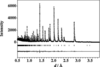Figure 1 Rietveld refinement pattern of 6H-type BaFeO3−δ at RT. Plus marks are the observed neutron diffraction intensities and the solid line shows the calculated patterns. Vertical marks below the profile indicate the positions of the Bragg reflections. The plot at the bottom is the difference between the observed and the calculated intensities.

The magnetization of BaFeO3−δ was measured using a SQUID on heating sample 1 after cooling it in zero field and applying a magnetic field of 150 Oe (1 Oe = 103 A m−1). Simultaneously, μ+SR experiments were performed with sample 1 at the Meson Science Laboratory (MSL) at the High Energy Accelerator Research Organization (KEK). TOF-SANS data for sample 2 were collected on the small- and wide-angle neutron diffractometer (SWAN) installed at the Neutron Science Laboratory (KENS) at KEK (Otomo et al., 1999).

### 3. Results

#### 3.1. Magnetic susceptibility

Fig. 2shows the temperature dependence of the magnetic susceptibility, χ (= M/H), of BaFeO3−δ, where M and H are the magnetization and applied magnetic field, respectively. Since χ increases drastically from 230 to 170 K, the χ−1 curve deviates from the straight line that would be expected from the Curie–Weiss law, as can be seen in the inset to Fig. 2. The decrease in the slope of the χ−1 curve would mean an increase in the effective number of Bohr magnetons, p, defined as p = gμB[S(S+ 1)]1/2, where g, μB and S are the g-factor (≃2), Bohr magneton and spin on the Fe ion, respectively. The drastic increase in p enables us to imagine the change of S due to the charge disproportionation of Fe4+ (Iga, Nishihara, Kido & Takeda, 1992). At around 170 K (= TN′), a sharp cusp appears on the χ spectrum. This is usually interpreted as an antiferromagnetic arrangement between Fe spins. This result is in good agreement with that reported by Iga, Nishihara, Katayama et al. (1992).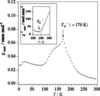Figure 2 Temperature dependence of the magnetic susceptibility of 6H-type BaFeO3−δ in a magnetic field of 150 Oe. The inset shows the reverse magnetic susceptibility as a function of T. The solid line indicates the Curie–Weiss law.

#### 3.2. μ+SR

Microscopic studies of the magnetism in BaFeO3−δ were carried out using μ+SR at temperatures between 15 and 298 K. μ+SR can be performed without applying any external field to samples. Figs. 3(a), (b) and (c) show the temperature dependence of the μ+SR time spectrum in zero magnetic field (ZF) at 125, 155 and 184 K.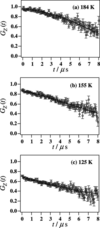Figure 3 Fitted μ+SR time spectra of 6H-type BaFeO3−δ in zero magnetic field at 184 K (a), 155 K (b) and 125 K (c). Grey marks are the observed μ+SR time spectra and solid lines are the calculated spectra. The GZ(t) values are normalized by GZ(0) at 298 K.

All ZF-μ+SR spectra were fitted as follows. Below 125 K, the exponential decay was expressed as a simple exponential relaxation function,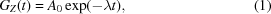where t, λ and A0 are the time, muon spin relaxation rate and initial asymmetry at t = 0. Above 184 K, the following relaxation function was used in analyses: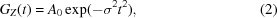where σ is the muon spin relaxation rate. This Gaussian decay indicates that the muons feel the almost static nuclear dipolar fields originating from the nuclei, because we could decouple the internal field with a longitudinal field (LF) of 70 G (1 G = 10−4 T) at 298 K. In the intermediate temperatures between 125 and 184 K, we assumed that the muon spin relaxation has two components: an exponential and a Gaussian decay, that is,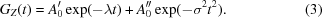It is noteworthy that the initial asymmetry is represented as A0 = A0′ + A0′′ at t = 0. As shown in Fig. 3, the experimental data could be fitted well by the above model. The λ and σ values converged at between 0.04 and 0.13 µs−1 at all temperatures.

Fig. 4shows A0 as a function of T. A0 rapidly decreases at around 170 K, therefore the Fe spin fluctuations become slow below 170 K. This result is consistent with that of the magnetization, showing the antiferromagnetic like Fe spin ordering at TN′. Below 120 K, A0 maintains a constant value of 0.67. It is most likely that Fe spins completely order below 120 K, and this result is in good agreement with that of the previous TOF-NPD experiments (TN ≃ 130 K) (Mori et al., 2003).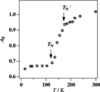Figure 4 Temperature dependence of the initial asymmetry, A0. The two arrows indicate the magnetic transition temperatures TN′ and TN.

#### 3.3. TOF-SANS

We carried out TOF-SANS experiments at temperatures between 4 and 200 K. Fig. 5shows neutron scattering intensities, I(Q), at 4, 130 and 200 K, where Q is the modulus of the scattering vector [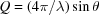, where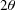is the scattering angle and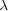is the wavelength]. In the low-Q region the profiles exhibit Q−4 dependence due to the grain boundary effect of the powder sample. As can be seen in Fig. 5(c), a magnetic Bragg peak of the 00δm reflection is observed at around Q ≃ 0.2 Å−1. The propagation vector is parallel to the 〈001〉 direction and δm is 0.45c* at 50 K, as reported elsewhere (Mori et al., 2003). Furthermore, it was found that a broad diffuse scattering exists in Q regions between 0.2 and 0.3 Å−1 (indexed as MDS in Fig. 5). The broad diffuse scattering originates from magnetic domains with a short-range correlation, because the X-ray powder diffraction pattern showed no such broad diffuse scattering. Here, we stress that the magnetic diffuse scattering and 00δm magnetic reflection appear simultaneously below 130 K, namely the short-range magnetic domains coexist with the long-range antiferromagnetic ordering phase.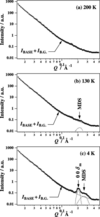Figure 5 SANS spectra of 6H-type BaFeO3−δ at 200 K (a), 130 K (b) and 4 K (c). Plus marks are the observed SANS intensities and the solid lines are the calculated ones. Three types of broken lines show the magnetic Bragg peak (00δm), the magnetic diffuse scattering (MDS) and IBASE + IB.G., which is the base function plus the constant background (see text).

To examine the details of the short-range magnetic ordering, SANS data were fitted for the Q region between 0.01 and 0.5 Å−1 through the following process. Firstly, the SANS spectrum at 200 K was represented by (see Fig. 5a)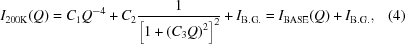where IB.G. is the constant background, and C1, C2 and C3 are coefficients. The second term on the right-hand side of equation (4)is given by the Debye–Bueche formula (Debye & Bueche, 1949). If IBASE scarcely depends on temperature, the C1 to C3 values obtained at 200 K can be used for SANS data at other temperatures. Considering the magnetic scattering contribution, the SANS spectra below 200 K were described by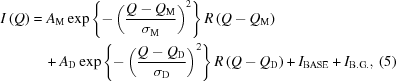where the first and second Gaussian terms correspond to the 00δm reflection at QM and magnetic diffuse scattering at QD, respectively. R(QQM) and R(QQD) indicate the Gaussian resolution function of SWAN, where its resolution, ΔQ/Q, is about 0.05 at around 0.2 Å−1 on the medium-angle detector bank (Otomo et al., 1999). As shown in Fig. 5, excellent fits between the observed and calculated patterns were obtained for all SANS data.

Fig. 6shows the intensities of the 00δm magnetic reflection and magnetic diffuse scattering versus T. All magnetic intensities are normalized by that of the 00δm reflection at 4 K. The intensity of the 00δm reflection gradually decreases with increasing T, and then the 00δm reflection completely disappears above 130 K. Thus, it could be confirmed that the Néel temperature for the antiferromagnetic ordering with a long-range correlation is ~130 K (= TN). In contrast, the magnetic diffuse scattering remains even above 130 K. The peak position of the magnetic diffuse scattering was estimated as QD ≃ 0.26 Å−1 at 4 K, which is obviously larger than that of the 00δm reflection (QM ≃ 0.2 Å−1). The average size of the magnetic domains at 4 K was estimated as ~124 Å on the basis of the Scherrer equation using the full width at the half maximum, FWHM = (4 ln 2)1/2σM ≃ 0.09 Å−1. Note that the magnetic diffuse scattering intensity rises rather than decreases with increasing T at around 120 K. We expect the unusual temperature dependence of the magnetic intensity to be an effect of the critical scattering owing to the antiferromagnetic ordering with long-range correlation, as shown in FexMn1−xTiO3, which shows a re-entrant spin-glass transition (Yoshizawa et al., 1987, 1989; Ito, 2000).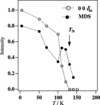Figure 6 Temperature dependence of the integrated magnetic intensities for the 00δm reflection and the magnetic diffuse scattering (MDS). Open and filled circles correspond to the 00δm reflection and the MDS, respectively. An arrow indicates the long-range antiferromagnetic ordering temperature, TN ≃ 130 K.

### 4. Discussion

TOF-SANS, magnetization and μ+SR experiments revealed the detailed magnetic state in 6H-type BaFeO3−δ at low temperature. With increasing T from 4 K to RT, the magnetic state changes in three stages: the mixed magnetic phase (the long-range antiferromagnetic ordering and short-range magnetic ordering), the short-range magnetic ordering phase and the paramagnetic phase. A schematic illustration is given in Fig. 7. Interestingly, similar behaviour is observed in another charge disproportionation compound, Sr3Fe2O7−δ, in which the short-range magnetic domains are formed above TN (Mori et al., 2001). Therefore, the relation between the complicated magnetic behaviour and charge disproportionation phenomenon would be worth studying. In the case of 6H-type BaFeO3−δ, the Fe4+ concentration decreases linearly below 170 K, as mentioned in §1. When the charge disproportionation of Fe4+ occurs locally, two kinds of Fe spins such as S = 5/2 on Fe3+ (t2g3eg2) and S = 3/2 on Fe5+ (t2g3eg0) instead of S = 4/2 on Fe4+ (t2g3eg1) should be produced in addition to S on Fe3+ arising from the oxygen deficiency. Even though in our TOF-SANS experiments the magnetic structure in the magnetic domains could not be determined from only one magnetic diffuse scattering peak, it is most likely that the appearance of the magnetic domains is caused by the spin-glass ordering, because a spin-glass-like large thermal hysteresis was found in the temperature dependence of the magnetization in 6H-type BaFeO3−δ (Iga, Nishihara, Katayama et al., 1992). Presumably, the randomly distributed frustrations, the size of which is about 124 Å, are created by the competition of the magnetic interaction between Fe spins with different S in the local region, reflecting the charge disproportionation of Fe4+. On the other hand, long-range antiferromagnetic ordering with the propagation vector parallel to the 〈001〉 direction would rapidly occur over the whole region except the domains with the spin-glass formation at around 130 K. It seems that the mixed magnetic phase can stably exist during the charge disproportionation process below 130 K.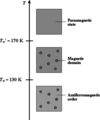Figure 7 Schematic illustration of aspects of the magnetic state depending on T for 6H-type BaFeO3−δ.

### 5. Conclusion

In this work, a magnetic diffuse scattering arising from magnetic domains with a short-range correlation was found in the TOF-SANS spectrum of 6H-type BaFeO3−δ at low temperature. The size of the magnetic domains was estimated as ~124 Å. Furthermore, the results obtained from magnetization and μ+SR experiments lead to the important conclusion that the magnetic domains appear below 170 K. The TOF-SANS experiments also demonstrated the existence of an antiferromagnatic ordering with a long-range correlation below 130 K. The long-range antiferromagnetic ordering coexists with the magnetic domains below 130 K. Thus, two different types of magnetic states exist in 6H-type BaFeO3−δ at low temperature, and this result explains the discrepancy of the Néel temperatures between three measurement techniques: magnetization, TOF-NPD and Mössbauer measurements.

### References

© International Union of Crystallography. Prior permission is not required to reproduce short quotations, tables and figures from this article, provided the original authors and source are cited. For more information, click here.JOURNAL OFAPPLIEDCRYSTALLOGRAPHY
ISSN: 1600-5767
Volume 40| Part s1| April 2007| Pages s501-s505
Follow J. Appl. Cryst.